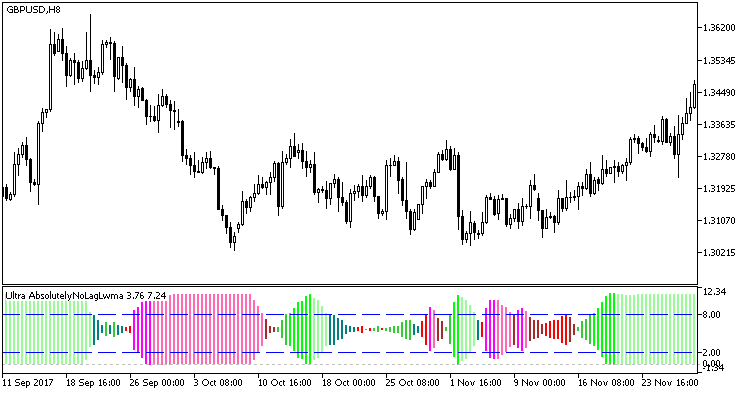Watch how to download trading robots for free

Interesting script?
So post a link to it -
let others appraise it

You liked the script? Try it in the MetaTrader 5 terminal# UltraAbsolutelyNoLagLwma - indicator for MetaTrader 5

Views:
525
Rating:
votes: 8
Published:
2018.07.09 10:30

This indicator is based on indicator AbsolutelyNoLagLwma and on the analysis of its multiple signal lines. Signal lines calculation algorithm is as follows. Any period value from the multitude of signal lines is calculated using arithmetic progression:

SignalPeriod(Number) = StartLength + Number * Step

The value of Number variable ranges from zero to StepsTotal. The obtained values ​​of the periods are added to the array of variables and are used at each tick of the indicator to get an array of the indicator's smoothed values. Based on that array, the directions of the current trend are calculated for each smoothing, and the numbers of positive and negative trends are found for the entire array the smoothed AbsolutelyNoLagLwma values.

The final numbers of positive and negative trends are smoothed and used as the indicator lines that form a color histogram displayed using the DRAW_COLOR_HISTOGRAM2 style class. Trend direction in this indicator is determined by the histogram color, while its power is determined by the histogram width.

To indicate the trend, four colors are used for each of the two trend directions: If the histogram values do not enter the overbought/oversold areas, then the indicator colors are darker, while they become lighter when the overbought/oversold levels are broken through.

### Inputs of the indicator are

```//+----------------------------------------------+
//| Indicator input parameters                   |
//+----------------------------------------------+
input uint FLength=7;                                // smoothing depth
input Applied_price_ IPC=PRICE_CLOSE_;              // price cconstant
//----
input Smooth_Method W_Method=MODE_JJMA;             // Smoothing method
input int StartLength=3;                            // Initial smoothing period
input int WPhase=100;                               // Smoothing parameter
//----
input uint Step=5;                                  // Period changing step
input uint StepsTotal=10;                           // Number of changes in the period
//----
input Smooth_Method SmoothMethod=MODE_JJMA;         // Smoothing method
input int SmoothLength=3;                           // Smoothing depth
input int SmoothPhase=100;                          // Smoothing parameter
//----
input uint UpLevel=80;                              // Overbought level in %%
input uint DnLevel=20;                              // Oversold level in %%
input color UpLevelsColor=Blue;                     // Overbought level color
input color DnLevelsColor=Blue;                     // Oversold level color
input STYLE Levelstyle=DASH_;                       // Levels style
input WIDTH  LevelsWidth=Width_1;                   // Levels thickness
```

Smoothing algorithms can be selected out of ten possible versions:

1. SMA - simple Moving Average;
2. EMA - exponential Moving Average;
3. SMMA - smoothed Moving Average;
4. LWMA - linear weighted moving average;
5. JJMA - adaptive averaging JMA;
6. JurX - ultralinear averaging;
7. ParMA - parabolic smoothing;
8. T3 - Tillson's multiple exponential smoothing;
9. VIDYA - smoothing with the use of Tushar Chande algorithm;
10. AMA - smoothing using Perry Kaufman's algorithm.

It should be noted that Phase type parameters for different smoothing algorithms have completely different meaning. For JMA it is an external Phase variable changing from -100 to +100. For T3 it is a smoothing ratio multiplied by 100 for better visualization, for the VIDYA it is a CMO oscillator period and for the AMA it is a slow EMA period. In other algorithms these parameters do not affect the averaging. For AMA fast EMA period is a fixed and equal to 2 by default value. The ratio of raising to the power is also equal to 2 for AMA.

The indicator uses SmoothAlgorithms.mqh library classes (copy it to <terminal_data_folder>\MQL5\Include). The use of the classes was described in detail in the article "Averaging Price Series for Intermediate Calculations Without Using Additional Buffers".Fig 1. Inicator UltraAbsolutelyNoLagLwma

Translated from Russian by MetaQuotes Software Corp.
Original code: https://www.mql5.com/ru/code/20975PCI

Indicator Phase Change Index - the market phase change indexInterceptor

Moving averages on charts M5, M15, H1 are analyzed. The presence of the iMA (Moving Average, MA) "fan" is checked simultaneously on all three charts (M5, M15, and H1).Exp_AbsolutelyNoLagLwma_X2

Trend-following trading system Exp_AbsolutelyNoLagLwma_X2 based on the signals of two indicators AbsolutelyNoLagLwmaColorJFatl_x2_cloud_HTF

Two indicators ColorJFatl from different timeframes, the area between lines being filled with a cloud, the color of which corresponds with the trend direction on the market